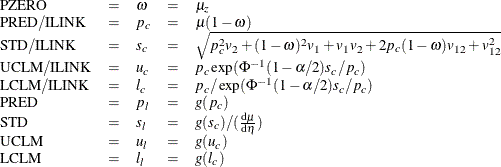# The PLM Procedure

### Scoring Data Sets for Zero-Inflated Models

The PLM procedure can score new observations for zero-inflated models with the SCORE statement. If you specify the ILINK option, the computed statistics are for estimated counts.

In the following formula,is the design row for covariates that correspond to the the Poisson or negative binomial component,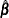is the column vector of the fitted regression parameters;is the design row for covariates that correspond to the zero inflation component,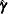is the column vector of the fitted regression parameters; g and g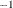are the link and inverse link functions for the Poisson or negative binomial component,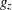and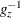are the link and inverse link functions for the zero inflation component;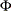is the standard normal cumulative distribution function andis the nominal significance level. Let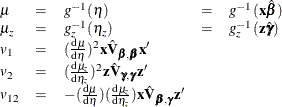The formula for statistics in the SCORE statement for zero-inflated models are listed as follows.